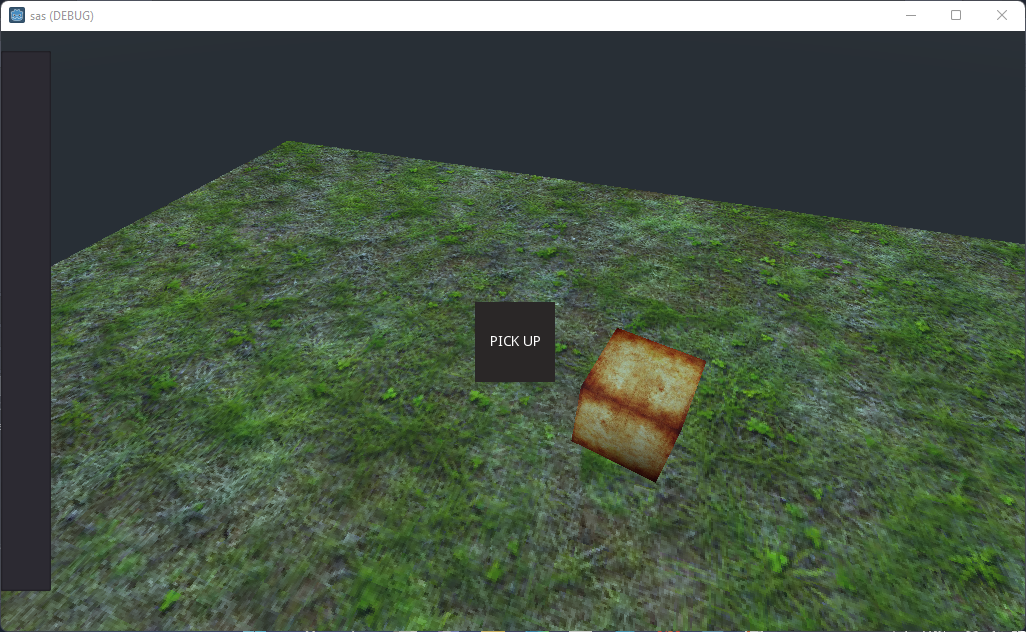+1 vote

So i'm using a kinematic body 3D nd i'm changing its movement direction using mouse rotation, by getting the mouse's basis.x nd basis.z, however when i try to calculate the distance between my player (kinematic body 3d) nd a softbody it doesn't work well, i've applied the formula sqrt(pow(x2-x1,2)+pow(y2-y1,2)+pow(z2-z1,2)) but it doesnt work well

it only works when iam in certain side, if i move to the other side it doesnt workthis my code for calculating the distance:

``````extends Spatial
``````

func physicsprocess(delta):
var x=\$KinematicBody.translation.x-\$SoftBody.translation.x
var z=\$KinematicBody.translation.z-\$SoftBody.translation.z
var y=\$KinematicBody.translation.y-\$SoftBody.translation.y
if (sqrt(pow(x,2)+pow(z,2)+pow(y,2))<2):
\$Control.visible=true
if Input.isactionjustpressed("uiaccept"):
else:
\$Control.visible=false

EDIT:
i want to let u guys know that i've found the reason of why the distance isnt calculated well, the reason is that both player nd softbody doesnt start at the same point, they both start with 0 coordinates but not at the same point, so now i just hav to find a way to get pass that

Godot version 3.5.1
in Engine
edited

i want to let u guys know that i've found the reason of why the distance isnt calculated well, the reason is that both player nd softbody doesnt start at the same point, they both start with 0 coordinates but not at the same point, so now i just hav to find a way to get pass that

+1 vote

Using `global_position` to calculate distances is much simpler.

``````var distance = abs(\$KinematicBody.global_position - \$SoftBody.global_position)
``````

The abs() function makes sure you don't get negative values as distances can not be negative.
Also am not sure you need that complicated looking formula just to calculate the distance between two objects.

by (2,017 points)

i've tried using position as i saw in the docs, it tells me that position doesnt exist in 3d, nd i tried global_position as u suggested nd its the same thing

+1 vote

``````var distance = global_transform.origin.distance_to(\$SoftBody.global_transform.origin)